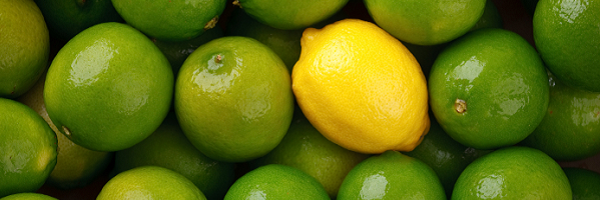# DifferencesChoose any three whole numbers, find the differences between them all, and find the product of the differences.

For example, if your three whole numbers are $7$, $4$ and $12$, the differences are:

$12 - 7 = 5$
$12 - 4 = 8$
$7 - 4 = 3$

The product of the differences is $3\times 5\times 8 = 120$

Try a few examples.

What do you notice?
Can you explain what you've noticed?

Now choose any four whole numbers, find the differences between them all, and find the product of the differences.

For example, if your four whole numbers are $7$, $4$, $12$ and $6$, the differences are:

$7-4 = 3$
$12-7 = 5$
$7-6 = 1$
$12-4 = 8$
$6-4 = 2$
$12-6 = 6$

The product of the differences is $3\times 5\times 1\times 8\times 2\times 6 = 1440$

Try a few examples.

What do you notice?
Can you explain what you've noticed?

### If we think of the three

If we think of the three numbers as A, B and C. There are 4 different option as to whether the gaps of the 3 number are odd or even.

We could have A - B even then B - C odd or even, which is 2 options.
Then we could have A - B odd and B - C even or odd. That's another two.

As we know that even-even=even, even-odd=odd and odd-odd=even...

For our first combination AB-even BC-odd which would mean AC would be odd, and an even times an odd times an odd is even, therefore for combination 1 the answer would always be even.

For combination 2 AB-even BC-even which would make AC also even, and an even times an even times an even is always even, therefore for combination 2, the answer would also always be even.

For combination 3 we had AB-odd BC-odd which would make AC even, and an odd times an odd times an even is an even, therefore for combination 3, the answer would always be even.

For combination 4 we had AB-odd BC-even which would make AC be odd, and an odd times an odd times an even make an even therefore for combination 4 the answer would always be even.

C1-EVEN
C2-EVEN
C3-EVEN
C4-EVEN

Therefore for any three whole numbers the product of their differences will ALWAYS be even.

### If we think of the three

Can you now say what happens when we consider the product of the differences of four numbers?

### If we think of the 4 numbers

If we think of the 4 numbers as A, B, C, D.

There will always be an even number in the differences therefore the answer will always be even. I'll show you how-
Let's call (B - A) 'W'
Let's call (C - B) 'H'
And let's call (C - A) 'U'

All the possible options of W and H being odd or even are:
1) W-ODD H-EVEN
2) W-ODD H-ODD
3) W-EVEN H-EVEN
4) W-EVEN H-ODD

The only possible option here without an even difference already is option 2, and for option 2 'U' would be even (due to the fact that C and A will either both be even or both be odd).

Therefore without even taking the fourth number into consideration, we can tell that the product of all the differences is always even. (because we only need one even in the differences, as anything times an even is even!)

Using this information we can now determine that for any given sequence of numbers above 2, the product of their differences will always be even!! :-)

### Four

The product of the first three number differences is even therefore the product of the differences of four+ numbers wil also be even, because anything times an even will always be even. So no matter how many numbers there are (over 3) the product of the differences will always be even.

### Four

Is the product of the differences of four numbers a multiple of any other numbers?

### Five

To work this out we have to work out the minimum number of even differences there can be:
If the minimum is 2 then the product of the differences has to be a multiple of 4,
if the minimum is 3 then the product of the differences has to be a multiple of 8,
if the minimum is 4 then the product of the differences has to be a multiple of 16,...

What I did to work this out was:
I made the gaps between the five numbers all odd. (In a sequence a, b, c, d, e. a-b=odd, b-c=odd, etc.)
This then...

... caused a-c, b-d and c-e to be even (odd+odd=even)
a-e would also be even (odd+odd+odd+odd=even)
This would also make a-d odd and b-e odd.

Therefore the minimum number of even numbers in the differences would be 4.

So for a sequence of 5 numbers the product of all their differences would be at least a multiple of 16.

### Five

Do you get the same result if the gaps between the five numbers are not all odd?

Is the product of the differences of five numbers a multiple of any other numbers?